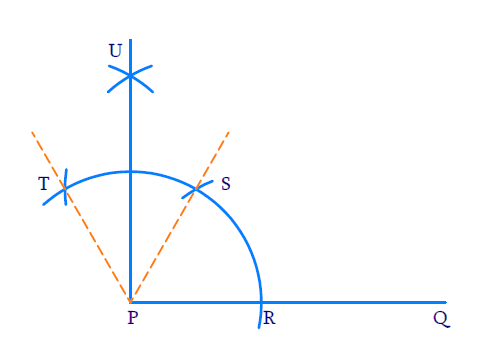# Ex.11.1 Q1 Constructions Solution - NCERT Maths Class 9

Go back to  'Ex.11.1'

## Question

Construct an angle of $$90^\circ$$ at the initial point of a given ray and justify the construction.

Video Solution
Constructions
Ex 11.1 | Question 1

## Text Solution

Reasoning:

We need to construct two adjacent angles each of $$60$$ degrees and bisect the second one to construct $$90$$ degree.

Steps of Construction:(i) Draw ray $$PQ$$

(ii) To construct $$60^{\circ}$$ angle. Draw an arc of any radius with $$P$$ as center intersecting $$PQ$$ at $$R$$ with $$R$$ as center and same radius draw an arc intersecting the previous arc at $$S$$. \begin{align}\angle {SPQ}=60^{\circ}\end{align}

(iii) To construct adjacent $$60^{\circ}$$ angle. With $$S$$ as the center and same radius as before intersecting the initial arc at $$T$$ $$\angle {TPS}$$ will be $$60^\circ$$

(iv) To bisect $$\angle {TPS} :$$

With $$T$$ and $$S$$ as centers and same radius as before draw two arcs to intersect each other at $$U$$. \begin{align}\angle {UPS}=\frac{1}{2} \angle {TPS}=30^{\circ}\end{align}

(v)  Join $$P$$ and $$U$$ to get an angle of $$60^{0}$$ at initial point $$P$$.

\begin{align} \angle {UPQ} &=\angle {UPS}+\angle {SPR} \\ &=30^{\circ}+60^{\circ} \\ &=90^{\circ} \end{align}

Learn from the best math teachers and top your exams

• Live one on one classroom and doubt clearing
• Practice worksheets in and after class for conceptual clarity
• Personalized curriculum to keep up with school